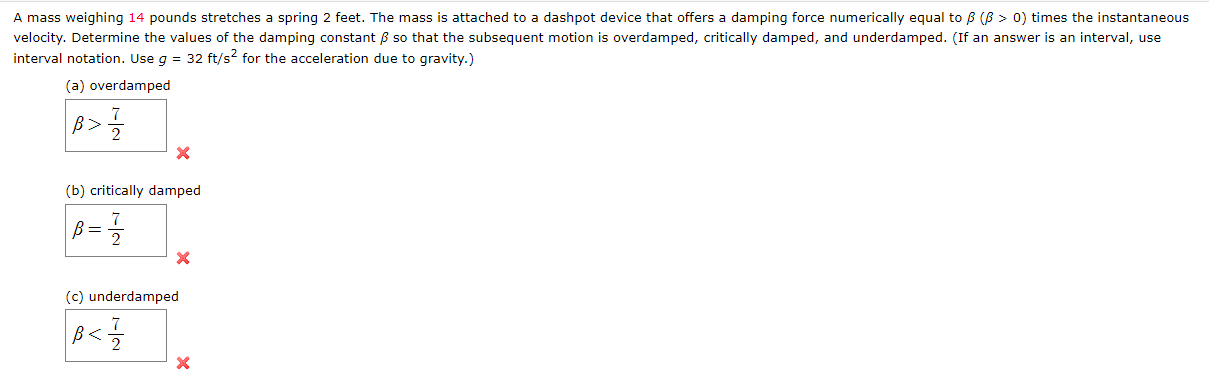# Question Solved1 AnswerA mass weighing 14 pounds stretches a spring 2 feet. The mass is attached to a dashpot device that offers a damping force numerically equal to β (β > 0) times the instantaneous velocity. Determine the values of the damping constant β so that the subsequent motion is overdamped, critically damped, and underdamped. (If an answer is an interval, use interval notation. Use g = 32 ft/s2 for the acceleration due to gravity.) (a) overdamped (b) critically damped (c) underdamped A mass weighing 14 pounds stretches a spring 2 feet. The mass is attached to a dashpot device that offers a damping force numerically equal to B (B > 0) times the instantaneous velocity. Determine the values of the damping constant B so that the subsequent motion is overdamped, critically damped, and underdamped. (If an answer is an interval, use interval notation. Use g = 32 ft/s2 for the acceleration due to gravity.) (a) overdamped |B> 1 / 2 (b) critically damped B= 11 / 1 X (c) underdampedA mass weighing 14 pounds stretches a spring 2 feet. The mass is attached to a dashpot device that offers a damping force numerically equal to β (β > 0) times the instantaneous velocity. Determine the values of the damping constant β so that the subsequent motion is overdamped, critically damped, and underdamped.

(If an answer is an interval, use interval notation. Use g = 32 ft/s2 for the acceleration due to gravity.)

(a) overdamped
(b) critically damped
(c) underdampedTranscribed Image Text: A mass weighing 14 pounds stretches a spring 2 feet. The mass is attached to a dashpot device that offers a damping force numerically equal to B (B > 0) times the instantaneous velocity. Determine the values of the damping constant B so that the subsequent motion is overdamped, critically damped, and underdamped. (If an answer is an interval, use interval notation. Use g = 32 ft/s2 for the acceleration due to gravity.) (a) overdamped |B> 1 / 2 (b) critically damped B= 11 / 1 X (c) underdamped
More
Transcribed Image Text: A mass weighing 14 pounds stretches a spring 2 feet. The mass is attached to a dashpot device that offers a damping force numerically equal to B (B > 0) times the instantaneous velocity. Determine the values of the damping constant B so that the subsequent motion is overdamped, critically damped, and underdamped. (If an answer is an interval, use interval notation. Use g = 32 ft/s2 for the acceleration due to gravity.) (a) overdamped |B> 1 / 2 (b) critically damped B= 11 / 1 X (c) underdamped